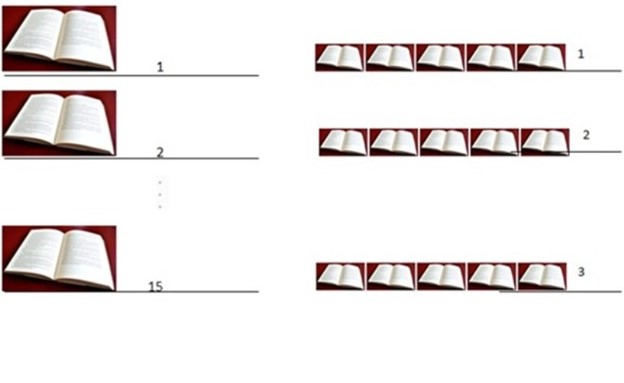# What is Modulation in Wireless Communications?

## Overview of Modulation in Wireless Communications

Like bidding in auction, telecom service providers also auction frequency bands. Huge investments are made while bidding even smaller bands- say a few megahertz. Bands in the very basic sense mean the 'air' around us. Air is a wireless ‘channel’/ ‘transmission medium’ that carries our data confined the electric and magnetic fields of the electromagnetic waves.

Therefore, bands mean the frequency ranges over which each service provider renders services to its subscribers. Since a huge amount of money is invested, effective utilization needs to be ensured. Technically, we need to use 'effective modulation techniques' for this purpose.

Without expanding the existing bandwidth, we can increase the data rate by modulating the user’s data strategically.

## Concept of Modulation

Modifying the way a user’s data is transmitted is known as modulation. Modulation does not alter any data of the user’s signal but only modifies the manner in which the data is transmitted. Modulation techniques can achieve increased data rates by transmitting more number of bits per symbol for a given symbol duration. Some popular modulation schemes include M-ary (4-PSK, 64-PSK and so on) modulation scheme and multi-carrier modulation scheme (OFDM and its variants/extensions).

Modulation does not alter any data of the user’s signal but
only modifies the manner in which the data is transmitted.

The existing bandwidth can’t be increased all the time to a great extent to
achieve high data rates and in such cases, modulation techniques come handy.

## Modulation - Bit rate and Symbol rate

We aim to maximize the bit rate (or data rate) of the communication system without expanding the existing bandwidth allocated for the application. Bit rate and Symbol rate are two important performance parameters for any communication link.

Bit rate specifies the number of bits transmitted per second while Symbol rate specifies the number of symbols transmitted per second.

Modulation techniques aim to increase the bit rate and subsequently the symbol
rate of the system by encoding multiple bits of data in a symbol and transmitting
the same in a given symbol duration.

## Numeric example

For a communication system, the 4 bits are encoded per symbol and four symbols are transmitted per second. Compute the bit rate of the system

Soln. Multiple bits are encoded per symbol to increase the bit rate. Here, 4 bits of data are encoded per symbol and 4 symbols are transmitted per second. Therefore, the bit rate is found using the expression given below −

$$Bit\:rate(\frac{bits}{second})=\frac{bits}{symbol}\times\:\frac{symbols}{second}=4\frac{bits}{symbol}\times\:4\frac{symbols}{second}$$

By substituting the known values, we can find the bit rate of the system to be 16 bits/second.

## Example - Understanding Modulation Concept in Detail

A student has 15 chapters to complete. The student takes 15 days to complete these chapters if he/she reads at the rate of a chapter a day. If he/she were to read five chapters a day, the student can complete the entire set in 3 days.

This is analogous to the number of bits encoded in a symbol. If more number of bits is encoded in a symbol, the bandwidth requirement reduces. However, this does burden the receiver (or sophisticated receivers are required). Coming back to the example- if the student reads five chapters a day, he/she only requires three days to complete the entire set. The remaining 12 days can be utilized for doing something else. The 'days' are analogous to 'bandwidth'. The remaining 'bandwidth' may be utilized by some other application that is bandwidth-hungry, like a high-resolution video clip.

Number of chapters studied in a day = Number of bits encoded per symbol